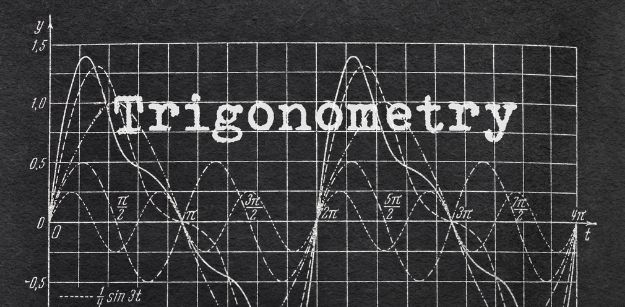# Tactics for Trigonometry for CBSE Class 10 Maths

Trigonometry or the study of triangles is a concept that is introduced freshly in CBSE class 10 maths syllabus. It involves the study of functions of angles and their application to calculations. Trigonometry can get a bit overwhelming and tricky for the students because it is loaded with formulas, ratios, functions, identities etc. There are a lot of new terms that they need to understand and once they do, they will find that the concept is really interesting and problems are fun to solve. Moreover, applications of trigonometry are also found in engineering, astronomy, physics and architectural designs.### Let us begin by recalling certain facts about the triangles that are important in Trigonometry:

• The sum of all 3 angles of a triangle is 180 degrees.
• The sum of the length of two sides of a triangle is greater than the third side whereas the difference between the length of two sides is less than the third side’s length.
• The longest side of a triangle is equal to the largest angle and vice versa.
• Formula to calculate the area of a triangle is: 1\2 x base x height
• According to Heron’s formula, Area of the triangle is √s(s−a) (s−b) (s−c), where s is the semi perimeter and is calculated by the following formula: ½ (a + b + c) where a, b, c, are the sides of the triangle.
• Pythagoras Theorem: This is a familiar topic that students have already studied in previous classes. It is extremely useful in solving trigonometry problems. The theorem states that the sum of squares of the base and height of a right triangle is equal to the square of the hypotenuse

The formula is: (Perpendicular)2+(Base)2=(Hypotenuse)2
⇒(P)2+(B)2=(H)2

### Now let us get familiar with trigonometry ratios or the trigonometry functions:

• Sine (or Sin)

Formula: Perpendicular/Hypotenuse

• Cosine (or Cos)

Formula: Base/Hypotenuse

• Tangent (or Tan)

Formula: Sine / Cosine = Perpendicular/Base

• Cotangent (or Cot)

Formula: Base/Perpendicular

• Cosec (or Cosecant)

Formula: Hypotenuse/Perpendicular

• Sec (or Secant)

Formula: Hypotenuse/Base

You will notice here that all of the functions are interrelated. That is a property of trigonometry. While values for Sine, Cosine, Tangent, etc. all have their uses, they are most useful because of the relationships that exist between them.

Considering θ is the angle in a right-angled triangle, there are three important trigonometry identities:

• Sin ² θ + Cos ² θ = 1
• Tan 2 θ + 1 = Sec2 θ
• Cot2 θ + 1 = Cosec2 θ

Some important points to be remembered:

• It is always recommended to start from the more complex side to solve trigonometric identities because it is a lot easier to eliminate terms to make a complex function simple than to find ways to make a simple function complex.
• To standardize both sides of the trigonometric identity, for easy comparison of one side to another always express everything into sine and cosine.
• To avoid mistakes, missing steps always combine terms into a single fraction when there are 2 terms on one side and 1 term on the other side after making their denominators the same.
• Staring at a question for a longer period will not magically pop out any answer. Sometimes, what a question merely requires is the good old expansion, factorisation, simplification, and cancelling of terms.

The key to ace at trigonometry is to learn and memorise all the formulas and apply them in questions accordingly. Always take one step and observe it. One should practice trigonometry every day and try to relate it with real-life applications. Trigonometry is extensively used by scientists, mathematicians to find the values for angles or line segments.

To learn more about such tactics for trigonometry of CBSE class 10 maths syllabus or to know about other NCERT solutions for class 10 maths, CBSE syllabus for class 10 check out Learnflix educational app for students- one of the best math learning apps.

•
•
•
•
•
•
•
•